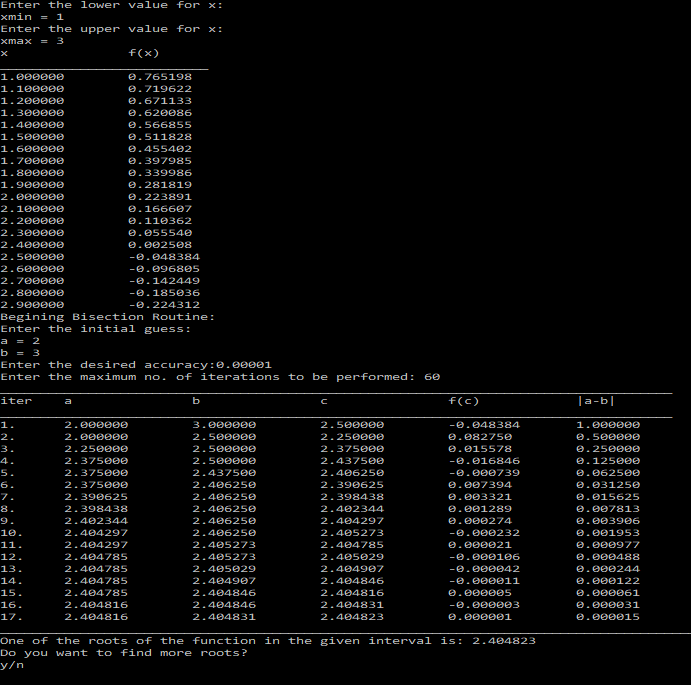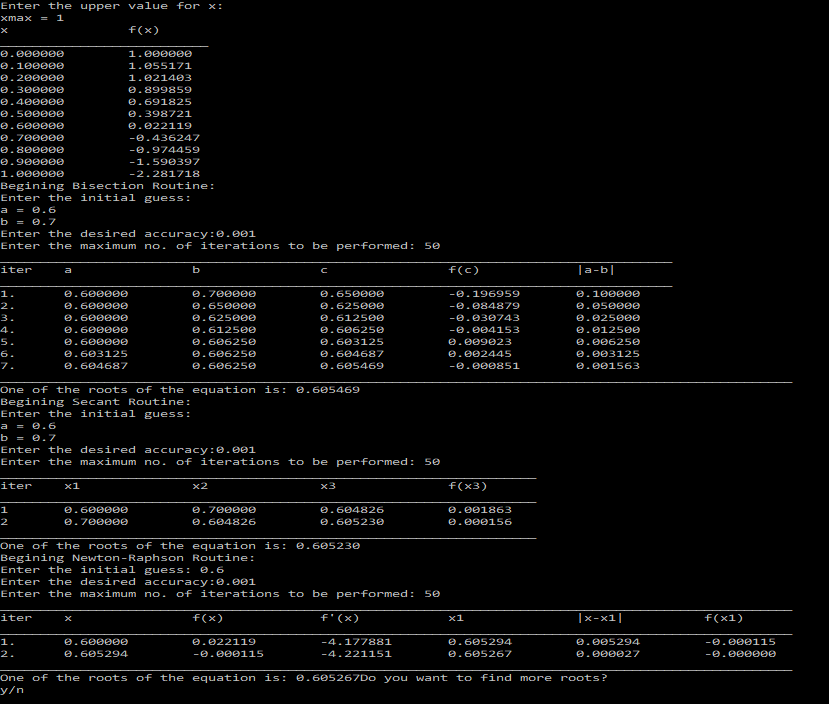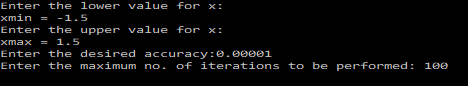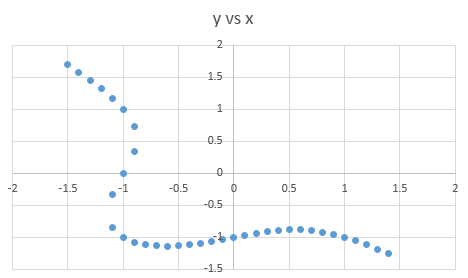# Numerical Root Finding Exercises – C Programming

I have already discussed about how to write C Programs for various Numerical Root Finding Methods like, Bisection Method, Secant Method and the Newton-Raphson Method.

I also discussed an application, where we evaluated the roots of the Chebyshev Polynomials using these methods.

In this post I will discuss a few more exercises on Numerical Root Finding.
I will be using a lot of previously written code, so it would be better if you read those posts first.

### Exercise 1

Using the series expansion for Jn(x),$J_n(x)=\left(\frac{x}{2}\right)^n\sum_{k=0}^{\infty}\frac{(-1)^k(\frac{x^2}{4})^k}{k!(n+k)!}$
find its two lowest positive roots for J0(x) to an accuracy of four decimal places.

### Solution

This is the series for the Bessel Functions of the first kind.
We have already discussed about how to create a program for Bessel Series.
So I’ll be re-using that code in the form of a function.
I will be using the Bisection Method to find the roots of the function.
I have already explained the code in a previous post, and I will be using that function as it is here.

The flow of the program would be something like this:
1. Ask the user to enter some range of x-values to tabulate the function, so that one can get an idea about where the root lies, and hence appropriate initial guesses can be given to the system.
2. Ask for initial guesses, tolerance value, max iterations, and print the root if found.

### PROGRAM:

/*************************************
******ROOT FINDING EXERCISE 2*********
*************************************/
#include<stdio.h>
#include<math.h>
//Function to calculate the factorial of an integer
double factorial(int n){
int i;
double fact=1;
for(i=n;i>=1;i--){
fact=fact*i;
}
return fact;
}
//A general function to find the sum of the Bessel series for a given n,x and accuracy(eps)
double Jn(double x, int n, double eps){
int k=1;
double t0,t1,sum,R;
t0=1/factorial(n);
sum=t0;
do{
R=-(x*x/4)/(k*(n+k));
t1=R*t0;
sum=sum+t1;
t0=t1;
k++;
}while(fabs(t1/sum)>eps);
sum=sum*pow(x/2.0,n);
return sum;
}
//Function that returns the value of J0(x) for a given x[WE NEED TO FIND THE ROOTS OF J0]
double J0(double x){
return Jn(x,0,0.00001);
}
/*The following function performs the bisection procedure and also prints the values of various variables at each iteration*/
double printBisection(double f(double x),double a, double b, double eps, int  maxSteps){
double c;
if(f(a)*f(b)<=0){
int iter=1;
/*Bisection Method begins that tabulates the various values at each iteration*/
printf("____________________________________________________________________________________\n");
printf("iter\ta\t\tb\t\tc\t\tf(c)\t\t|a-b|\n");
printf("____________________________________________________________________________________\n");
do{
c=(a+b)/2;
printf("%d.\t%lf\t%lf\t%lf\t%lf\t%lf\n",iter,a,b,c,f(c),fabs(a-b));
if(f(a)*f(c)>0){
a=c;
}
else if(f(a)*f(c)<0){
b=c;
}
iter++;

}while(fabs(a-b)>=eps&&iter<=maxSteps);
printf("___________________________________________________________________________________________________\n");
return c;
}
else{
printf("\nSorry! Either the root doesn't exist in the given interval or there are multiple roots in this interval.\nPlease enter a different set of guesses.\n");
return 9999;
}
}
main(){
//Let us first tabulate the function for a given range of x
double xmin, xmax;
printf("Enter the lower value for x:\nxmin = ");
scanf("%lf",&xmin);
printf("Enter the upper value for x:\nxmax = ");
scanf("%lf",&xmax);
double x;
printf("x\t\tf(x)\n");
printf("__________________________\n");
for(x=xmin;x<=xmax;x=x+0.1){
printf("%lf\t%lf\n",x,J0(x));
}
char choice='y';
while(choice=='y'){
//Begin Bisection Routine
printf("Begining Bisection Routine:\n");
double a,b,eps;
int maxSteps;
printf("Enter the initial guess:\na = ");
scanf("%lf",&a);
printf("b = ");
scanf("%lf",&b);
printf("Enter the desired accuracy:");
scanf("%lf",&eps);
printf("Enter the maximum no. of iterations to be performed: ");
scanf("%d",&maxSteps);
double root=printBisection(J0,a,b,eps,maxSteps);
//9999 is the error code returned by the bisection function if the given interval dosen't bracket the root or contains more than 1 root
if(root!=9999){
printf("One of the roots of the function in the given interval is: %lf",root);
}

printf("\nDo you want to find more roots?\ny/n\n");
scanf(" %c", &choice);
}
}


### OUTPUT:### Exercise 2

Find the roots, accurate to four decimal places, of the equation$e^x-5x^2=0$
in the range$-1 \leq x \leq1$.
by three iteration methods, that is Bisection, Secant and Newton-Raphson methods. In each case,
determine the number of iterations necessary to obtain the desired accuracy.

### SOLUTION:

In this problem, I would be using the functions(code) that I wrote previously for the three methods.
The program would first ask the user to enter the range of x to tabulate the function for that range, so that the user can get some idea about where the root lies and hence provide better initial guesses.
Then the program would run all the three methods(routines) one by one, and ask for the initial guesses, accuracy, etc. for each method.
To compare the three methods, one should enter the same initial guesses for all the three methods. However, since Newton-Raphson Method takes just one guess as opposed to the other two methods, so I will just use any one of those initial guesses.

### PROGRAM:

/*******************************************
******ROOT FINDING METHODS EXERCISE 1*******
*******************************************/
#include<stdio.h>
#include<math.h>

//Define the function whose root is to be determined
double f(double x){
return exp(x)-5*x*x;
}
//Define the derivative of the function(only needed for the Newton-Raphson Method)
double df(double x){
return exp(x)-10*x;
}
/*The following function performs the bisection procedure and also prints the values of various variables at each iteration*/
double printBisection(double f(double x),double a, double b, double eps, int  maxSteps){
double c;
if(f(a)*f(b)<=0){
int iter=1;
/*Bisection Method begins that tabulates the various values at each iteration*/
printf("____________________________________________________________________________________\n");
printf("iter\ta\t\tb\t\tc\t\tf(c)\t\t|a-b|\n");
printf("____________________________________________________________________________________\n");
do{
c=(a+b)/2;
printf("%d.\t%lf\t%lf\t%lf\t%lf\t%lf\n",iter,a,b,c,f(c),fabs(a-b));
if(f(a)*f(c)>0){
a=c;
}
else if(f(a)*f(c)<0){
b=c;
}
iter++;

}while(fabs(a-b)>=eps&&iter<=maxSteps);
printf("___________________________________________________________________________________________________\n");
return c;
}
else{
printf("\nSorry! Either the root doesn't exist in the given interval or there are multiple roots in this interval.\nPlease enter a different set of guesses.\n");
return 9999;
}
}
/*Secant Method Function that tabulates the values at each iteration*/
double printSecant(double f(double x), double x1, double x2, double eps, int maxSteps){
int iter=1;
double x3;
printf("___________________________________________________________________\n");
printf("iter\tx1\t\tx2\t\tx3\t\tf(x3)\n");
printf("___________________________________________________________________\n");
do{
x3=(x1*f(x2)-x2*f(x1))/(f(x2)-f(x1));
printf("%d\t%lf\t%lf\t%lf\t%lf\n",iter,x1,x2,x3,f(x3));
x1=x2;
x2=x3;
iter++;
}while(fabs(f(x3))>eps&&iter<=maxSteps);
printf("___________________________________________________________________\n");
return x3;
}
double printNR(double f(double x),double df(double x),double x1,double eps,double maxSteps){
double x;
int iter=1;
printf("___________________________________________________________________________________________________\n");
printf("iter\tx\t\tf(x)\t\tf'(x)\t\tx1\t\t|x-x1|\t\tf(x1)\n");
printf("___________________________________________________________________________________________________\n");
do{
x=x1;
if(fabs(df(x))>=0.000000001){
x1=x-f(x)/df(x);
printf("%d.\t%lf\t%lf\t%lf\t%lf\t%lf\t%lf\n",iter,x,f(x),df(x),x1,fabs(x-x1),f(x1));
iter++;
}
}while(fabs(x-x1)>=eps&&iter<=maxSteps);
printf("___________________________________________________________________________________________________\n");
return x1;
}
main(){
//Let us first tabulate the function for a given range of x
double xmin, xmax;
printf("Enter the lower value for x:\nxmin = ");
scanf("%lf",&xmin);
printf("Enter the upper value for x:\nxmax = ");
scanf("%lf",&xmax);
double x;
printf("x\t\tf(x)\n");
printf("__________________________\n");
for(x=xmin;x<=xmax;x=x+0.1){
printf("%lf\t%lf\n",x,f(x));
}
char choice='y';
while(choice=='y'){
//Begin Bisection Routine
printf("Begining Bisection Routine:\n");
double a1,b1,eps1;
int maxSteps1;
printf("Enter the initial guess:\na = ");
scanf("%lf",&a1);
printf("b = ");
scanf("%lf",&b1);
printf("Enter the desired accuracy:");
scanf("%lf",&eps1);
printf("Enter the maximum no. of iterations to be performed: ");
scanf("%d",&maxSteps1);
double root1=printBisection(f,a1,b1,eps1,maxSteps1);
if(root1!=9999){
printf("One of the roots of the equation is: %lf\n",root1);
}
//Begin Secant Routine
printf("Begining Secant Routine:\n");
double a2,b2,eps2;
int maxSteps2;
printf("Enter the initial guess:\na = ");
scanf("%lf",&a2);
printf("b = ");
scanf("%lf",&b2);
printf("Enter the desired accuracy:");
scanf("%lf",&eps2);
printf("Enter the maximum no. of iterations to be performed: ");
scanf("%d",&maxSteps2);
double root2=printSecant(f,a2,b2,eps2,maxSteps2);
printf("One of the roots of the equation is: %lf\n",root2);
//Begin Newton-Raphson Routine
printf("Begining Newton-Raphson Routine:\n");
double a3,eps3;
int maxSteps3;
printf("Enter the initial guess: ");
scanf("%lf",&a3);
printf("Enter the desired accuracy:");
scanf("%lf",&eps3);
printf("Enter the maximum no. of iterations to be performed: ");
scanf("%d",&maxSteps3);
double root3=printNR(f,df,a3,eps3,maxSteps3);
printf("One of the roots of the equation is: %lf",root3);

printf("Do you want to find more roots?\ny/n\n");
scanf(" %c", &choice);
}
}



### OUTPUT:### EXERCISE 3

The equation$f(x,y)=0$
defines y as an implicit function of x. As an example consider$f(x,y)=x^3+y^3+xy+1$
For any given x, this is a cubic equation in y; so y can be found by obtaining the roots (one or
three real roots) of this equation, say by secant method. Plot y as a function of x, for$-1.5 \leq x \leq 1.5$. If for some value of x there are three real roots,$(y1,y2,y3)$, plot all the three points$(x,y1); (x,y2); (x,y3)$. You may assume that$-2\leq y \leq y$

### SOLUTION

The above problem is a little tricky, and not so straight-forward as the prior ones.
Here, we are required to find all the real roots that exist. However, there is one help, that we are given that$-2\leq y \leq y$. So we have an idea about where to look for the roots.
I have used the Bisection Method to find the roots, as I found it to be the most suitable for this problem.
Now using the given hint about the possible values of y I need to give proper enough and suitable initial guesses to find the values of all the roots. To achieve this, I ran a loop from -2 to 2 and looked for the roots in small intervals of 0.5 width. So basically, for a given value of x, I used many initial guesses(both 0.5 apart) from -2 to 2. This way I was able to find all the real roots.
The program may appear to be extraordinarily long, but that is only because I have tried to pack everything into functions.

### PROGRAM:

/*************************************
******ROOT FINDING EXERCISE 3*********
*************************************/
#include<stdio.h>
#include<math.h>
double f(double x, double y){
return pow(x,3)+pow(y,3)+x*y+1;
}
/*The following function performs the bisection procedure and also prints the values of various variables at each iteration */
double printBisection(double x,double f(double x,double y),double a, double b, double eps, int  maxSteps){
double c;
if(f(x,a)*f(x,b)<=0){
int iter=1;
/*Bisection Method begins that tabulates the various values at each iteration*/
printf("____________________________________________________________________________________\n");
printf("iter\ta\t\tb\t\tc\t\tf(c)\t\t|a-b|\n");
printf("____________________________________________________________________________________\n");
do{
c=(a+b)/2;
printf("%d.\t%lf\t%lf\t%lf\t%lf\t%lf\n",iter,a,b,c,f(x,c),fabs(a-b));
if(f(x,a)*f(x,c)>0){
a=c;
}
else if(f(x,a)*f(x,c)<0){
b=c;
}
iter++;

}while(fabs(a-b)>=eps&&iter<=maxSteps);
printf("___________________________________________________________________________________________________\n");
return c;
}
else{
//printf("\nSorry! Either the root doesn't exist in the given interval or there are multiple roots in this interval.\nPlease enter a different set of guesses.\n");
return 9999;
}
}
/*Function definition for bisection procedure[Returns the root if found or 999 for failure]*/
double bisection(double x,double f(double x,double y),double a, double b, double eps, int maxSteps){
double c;
if(f(x,a)*f(x,b)<=0){
int iter=1;
/*Bisection Method begins that tabulates the various values at each iteration*/
do{
c=(a+b)/2;
if(f(x,a)*f(x,c)>0){
a=c;
}
else if(f(x,a)*f(x,c)<0){
b=c;
}
iter++;

}while(fabs(a-b)>=eps&&iter<=maxSteps);
return c;
}
else{
return 9999;
}
}

main(){
FILE *fp=NULL;
fp=fopen("rootFindingProblem3.txt","w");
double xmin, xmax,eps;
printf("Enter the lower value for x:\nxmin = ");
scanf("%lf",&xmin);
printf("Enter the upper value for x:\nxmax = ");
scanf("%lf",&xmax);
int maxSteps;
printf("Enter the desired accuracy:");
scanf("%lf",&eps);
printf("Enter the maximum no. of iterations to be performed: ");
scanf("%d",&maxSteps);
double x;
for(x=xmin;x<=xmax;x=x+0.1){
double y1,y2;
for(y1=-2;y1<=2;y1=y1+0.5){
y2=y1+0.5;
double root;
root=bisection(x,f,y1,y2,eps,maxSteps);
if(root!=9999){
fprintf(fp,"%lf\t%lf\n",x,root);
}
}
}
}


The above program will ask the user to enter the range of x values for which y is to be plotted. The program will then continue to find the value(s) of y for different x-values in the given range at an interval of 0.1.
The values are calculated upto a certain accuracy as desired by the user.
The program after executing fully, will create a file called 'rootFindingProblem3,txt'  containing the data-points to be plotted.
These can be plotted using softwares like Gnuplot and MS Excel.

### OUTPUT:### GRAPH:

Gnuplot Command: plot ‘./rootFindingProblem3.txt’

Excel:### EXERCISE 4:

Choosing equally spaced values of$t$ in$(0,\frac{2\pi}{\omega})$, solve the Kepler equation for$\psi$$\psi -\epsilon \psi-\omega t =0$
Use the solution to plot the orbit whose radial coordinates are given by$r= a(1-\epsilon \cos \psi)$$\cos \theta=\frac{\cos \psi -\epsilon}{1-\epsilon \cos \psi}$
Take$\omega=1.0$ ,$\epsilon =0.8$ and$a=2.0$. Remember that time$t$, is only a parameter. The equation has to be solved for each$t$ in the given interval. For each$t$, the initial value of$\psi$ can be chosen to be$t$.

### PROGRAM:

/*************************************
******ROOT FINDING EXERCISE 4*********
*************************************/
#include<stdio.h>
#include<math.h>
//Define the function whose root is to be determined
double f(double psi, double t){
//return the kepler's equation for: w=1, eccentricity=0.8
return psi-0.8*sin(psi)-t;
}
//Define the derivative of the function(only needed for the Newton-Raphson Method)
double df(double psi){
return 1-0.8*cos(psi);
}
/*Function that returns the root from Newton-Raphson Method*/
double rootNR(double t,double f(double x,double t),double df(double x),double x1,double eps,double maxSteps){
double x;
int i=1;
do{
x=x1;
if(fabs(df(x))>=0.000000001){
x1=x-f(x,t)/df(x);
i++;
}
}while(fabs(x-x1)>=eps&&i<=maxSteps);
return x1;
}
double r(double psi){
return 2*(1-0.8*cos(psi));
}
double coss(double psi){
return (cos(psi)-0.8)/(1-0.8*cos(psi));
}
double sinn(double psi){
return sin(acos(coss(psi)));
//return sqrt(1-coss(psi)*coss(psi));
}
main(){
FILE *fp=NULL;
FILE *fp1=NULL;
//File containing the data-points for the plot of Eccentric Anamoly(E) vs. Mean anamoly(wt)
fp=fopen("rootFindingProblem41.txt","w");
//File containing the data-points for the obital path
fp1=fopen("rootFindingProblem42.txt","w");
double t, eps;
int maxSteps;
printf("Enter the desired accuracy:");
scanf("%lf",&eps);
printf("Enter the maximum no. of iterations to be performed: ");
scanf("%d",&maxSteps);
for(t=0;t<=6.28;t=t+0.01){
double a=t;
double root;
root=rootNR(t,f,df,a,eps,maxSteps);
double temp;
temp=sinn(root);
if(t>3.14){
temp=-sinn(root);
}
//plot E(Eccentric anamoly for eccentricity=0.8) vs M(Mean anamoly, w=1)
fprintf(fp,"%lf\t%lf\n",t,root);
//plot the orbit
fprintf(fp1,"%lf\t%lf\n",r(root)*coss(root),r(root)*temp);
}
}


### REFERENCES:

The above problems have been taken from the Computer Programming & Numerical Analysis Manual by Dr. Shobhit Mahajan.[wpedon id="7041" align="center"]